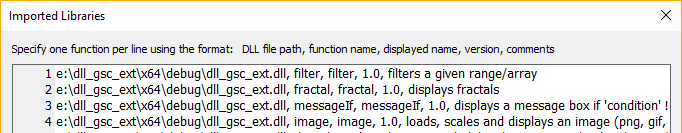## Adding functions in DLL libraries

Additional or modified functions in DLL libraries
Posts: 215
Joined: Tue Apr 20, 2010 11:58 pm

### Adding functions in DLL libraries

GS-Calc (ver. 17 and later) can be configured to use functions written by users in C/C++ as plain DLL libraries. Such functions can be even used as a replacement for the default built-in functions. They can accept and return all the argument types and additionally they can also return images displayed directly in worksheets or messages displayed after each update. What's important, using these functions GS-Calc retains the complete multicore support, the same speed and memory requirements (e.g. no extra arrays are allocated when passing ranges as arguments). You can add virtually any number of such functions.

Libraries are added by the Settings > Imported Function Libraries command as shown on the screenshot below. The internal function names must match the original C/C++ names. The displayed names are what is entered in worksheet cells and the only requirement is that they must be unique.The added functions must be declared as

Code: Select all

``typedef double(__cdecl *DLL_EXT_FUNCTION)(GSCalcArg *arg);``
where GSCalcArg is a structure enabling you to pass up to 15 arguments: numbers, strings and ranges/arrays.

Code: Select all

``````struct ArrayItem
{
uint8_t type;		// DLL_ARGT_EMPTY | DLL_ARGT_DOUBLE | DLL_ARGT_TEXT
uint8_t err_code;	// ERROR1_DIV_BY_ZERO...ERROR1_SYNTAX_ERROR
uint16_t col;		// [0, 4095]
uint32_t row;		// [0, 12582911]
double num;		// numeric cell
char text[MAXINPUT + 1];// text cell; max. 1024+NULL char. utf-8 string
};

typedef int(__cdecl *READ_ARRAY)(void *env, int array_index, ArrayItem *val);
typedef int(__cdecl *WRITE_ARRAY)(void *env, int array_index, ArrayItem *val);

typedef void* (__cdecl *MALLOC)(size_t s);
typedef void(__cdecl *MFREE)(void *_Memory);

struct GSCalcArg
{
uint8_t types;		// in: as in ArrayItem.type,  out: DLL_ARGT_EMPTY...DLL_ARGT_MESSAGE
uint8_t errors;		// ERROR1_DIV_BY_ZERO...ERROR1_SYNTAX_ERROR
double numbers;
char *strings;		// input (UTF-8) 0...14 strings are preallocated by GS-Calc and must not be overwritten;
// strings is used for output strings and already points to a MAXINPUT+1 char. temp. buffer
struct Dims
{
uint32_t cx;
uint32_t cy;
} array_dims;		// dimensions of the subsequent and grouped together range/array arguments passed from GS-Calc
struct
{
BYTE *data;		// DIB data if you return types = DLL_ARGT_IMAGE; must be (de-)allocated with memory_alloc/memory_free
size_t size;		// DIB data size
Dims dims;		// optional resizing when displaying the image (returned either as a DIB or a file path)
MALLOC memory_alloc;
MFREE memory_free;
} image;
WRITE_ARRAY write_array;	// returns ArrayItem.type or -1 if the out-of-memory condition occurs
void *env;			// internal data that must be passed back in read_array/write_array calls
};
``````
Indices 0...14 in types[] and errors[] in GSCalcArg represent all subsequent argument types and errors from left to right passed from GS-Calc.

Indices 0...14 in numbers[], strings[] and array_dims[] point to argument values after breaking them down into 3 groups (numbers, strings and ranges/arrays) so each group receives its own counter.

On output, the index 15 (DLL_ARGC_RET) is used to specify the returned type, error code and value. The types and errors are always set obligatorily.
The returned values are stored as follows:
• double number - the numbers value must be set accordingly.
• string (null terminated) - must be copied to the strings buffer (up to 1024+1 characters including NULL) preallocated by GS-Calc.
• array - the returned array is filled with the write_array function and array_index set to 15. The array_dims array size is determined automatically based on the col/row values passed to write_array. It can be overwritten if needed.
• image - either BYTE *data and size_t size in Image are set or the full file path is copied to strings

Examples:

Code: Select all

``````// messageIf(condition, text)
//
// arguments:
//			condition, message text
// returns:
//			if condition != 0, 'messageIf' displays 'text' as a message box after each update/recalculation
//			otherwise the message text is displayed in a cell as normal text

__declspec(dllexport) double __cdecl messageIf(GSCalcArg *arg)
{
uint8_t errorCode = 0;

if (arg->types != DLL_ARGT_DOUBLE && arg->types != DLL_ARGT_EMPTY || arg->types != DLL_ARGT_TEXT)
errorCode = ERROR1_INVALID_VALUE;
if (arg->types != DLL_ARGT_NONE)	// more than 2 arguments detected
errorCode = ERROR1_SYNTAX_ERROR;

if (!errorCode && arg->errors)
errorCode = arg->errors;
if (!errorCode && arg->errors)
errorCode = arg->errors;

if (!errorCode)
{
::strncpy(arg->strings[DLL_ARGC_RET], arg->strings, 1024);
arg->strings[DLL_ARGC_RET] = 0;
return arg->types[DLL_ARGC_RET] = (arg->numbers ? DLL_ARGT_MESSAGE : DLL_ARGT_TEXT);
}
else
{
arg->errors[DLL_ARGC_RET] = errorCode;
return arg->types[DLL_ARGC_RET] = DLL_ARGT_EMPTY;
}
}
``````

Code: Select all

``````// filter(range, column, number)
//
// arguments:
//			range - a range/array
//			column - column index (from 0 to the number of columns in 'range' - 1)
//			number - numeric value to perform equality check
// returns:
//			an array consisting of rows of 'range' containing 'number' in the specified column

__declspec(dllexport) double __cdecl filter(GSCalcArg *arg)
{
uint8_t errorCode = 0;

if (arg->types != DLL_ARGT_ARRAY || arg->types != DLL_ARGT_DOUBLE || arg->types != DLL_ARGT_DOUBLE)
errorCode = ERROR1_INVALID_VALUE;
if (arg->types != DLL_ARGT_NONE)
errorCode = ERROR1_SYNTAX_ERROR;

if (!errorCode && arg->numbers >= arg->array_dims.cx)
errorCode = ERROR1_INVALID_VALUE;
if (!errorCode && arg->errors)
errorCode = arg->errors;
if (!errorCode && arg->errors)
errorCode = arg->errors;
if (!errorCode && arg->errors)
errorCode = arg->errors;

ArrayItem x = { 0 };
int outRow = 0;

for (uint32_t inRow = 0; inRow < arg->array_dims.cy && !errorCode; ++inRow)
{
x.row = inRow;
x.col = static_cast<uint16_t>(arg->numbers);
if (arg->read_array(arg->env, 0, &x) == -1)
{
errorCode = x.err_code;
break;
}

bool match = false;
if (x.type == DLL_ARGT_DOUBLE)
match = (x.num == arg->numbers || x.err_code && x.err_code == arg->errors);
else if (x.type == DLL_ARGT_EMPTY)
match = (arg->numbers == 0);
else
errorCode = ERROR1_INVALID_VALUE;

if (match)
{
for (x.col = 0; x.col < arg->array_dims.cx && !errorCode; ++x.col)
{
x.row = inRow;
x.row = outRow;

// for best performance when writing to very large arrays, try to write in the left-to-right and top-to-bottom order

if (arg->write_array(arg->env, DLL_ARGC_RET, &x) == -1) // -1 means the out-of-memory condition or an invalid row/column
errorCode = x.err_code;
}
++outRow;
}
}

arg->errors[DLL_ARGC_RET] = (!outRow ? ERROR1_NULL_VALUE : 0);
return arg->types[DLL_ARGC_RET] = DLL_ARGT_ARRAY;
}
``````